Ex 13.2

Chapter 13 Class 10 Surface Areas and Volumes (Term 2)
Serial order wise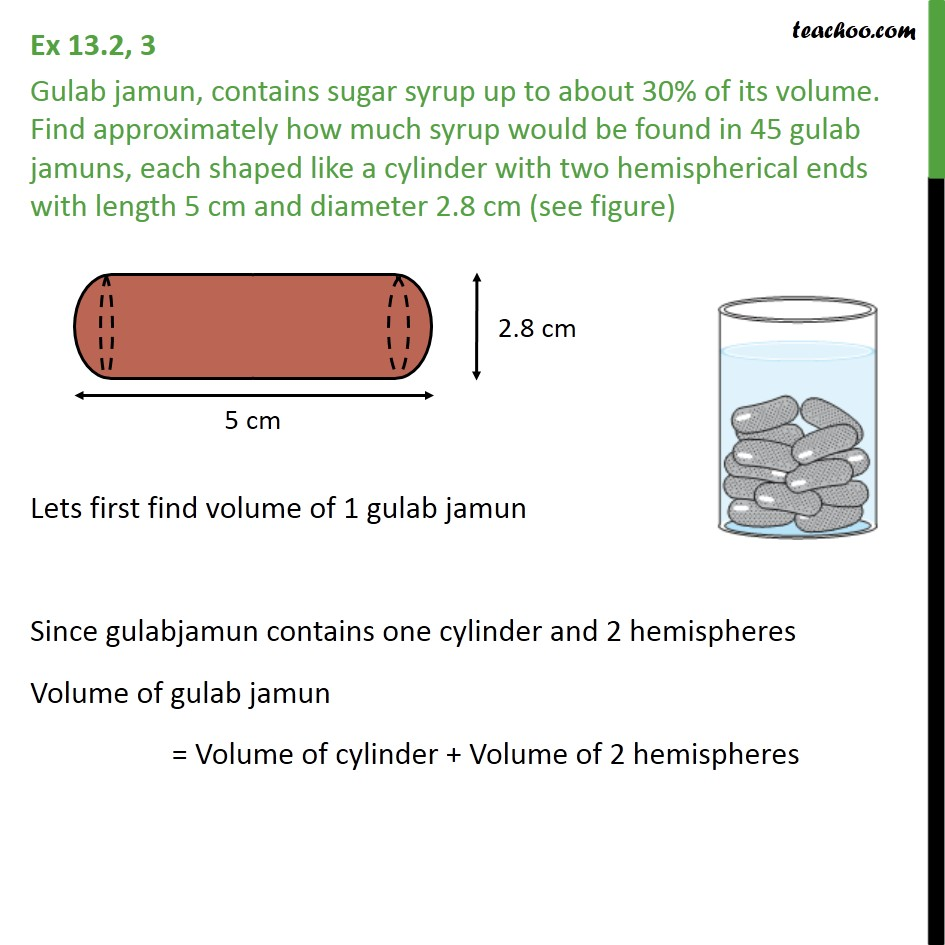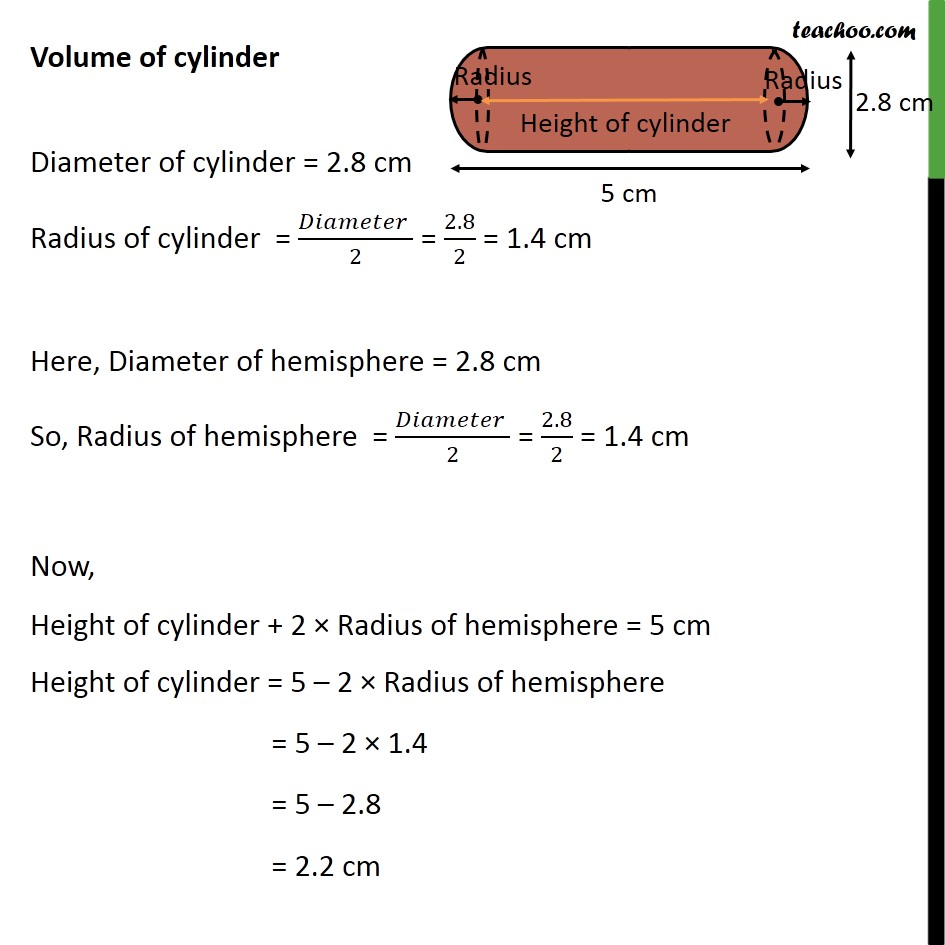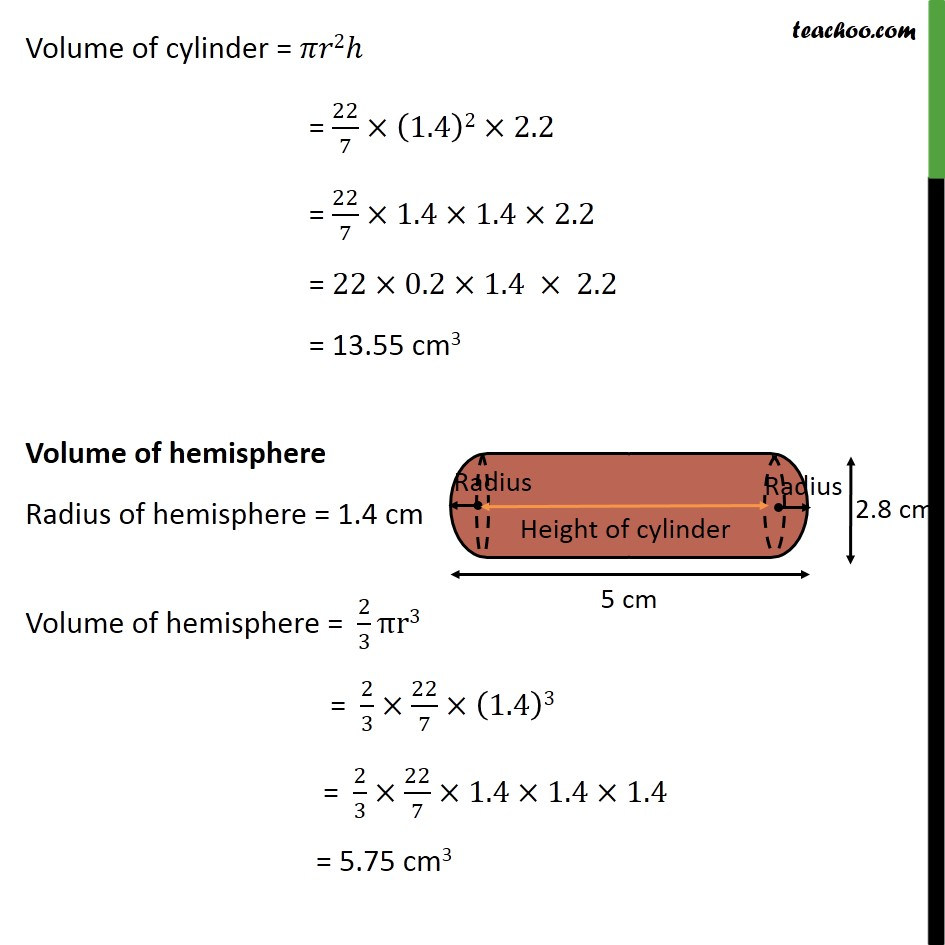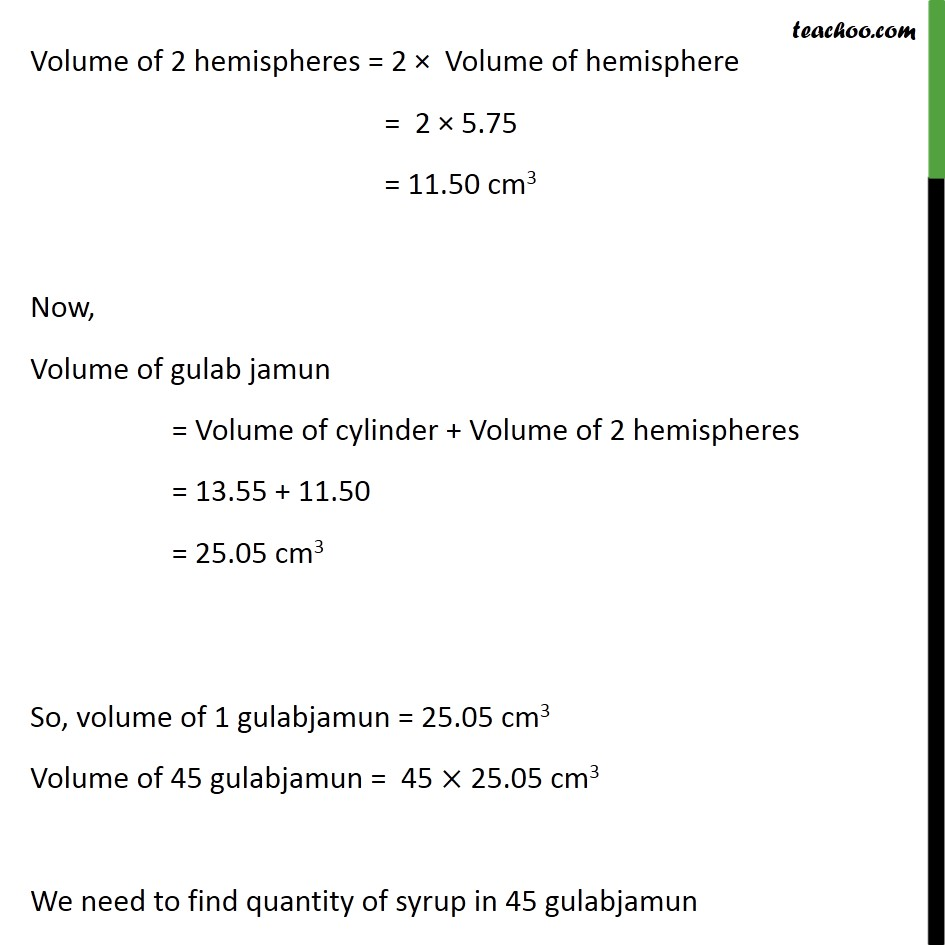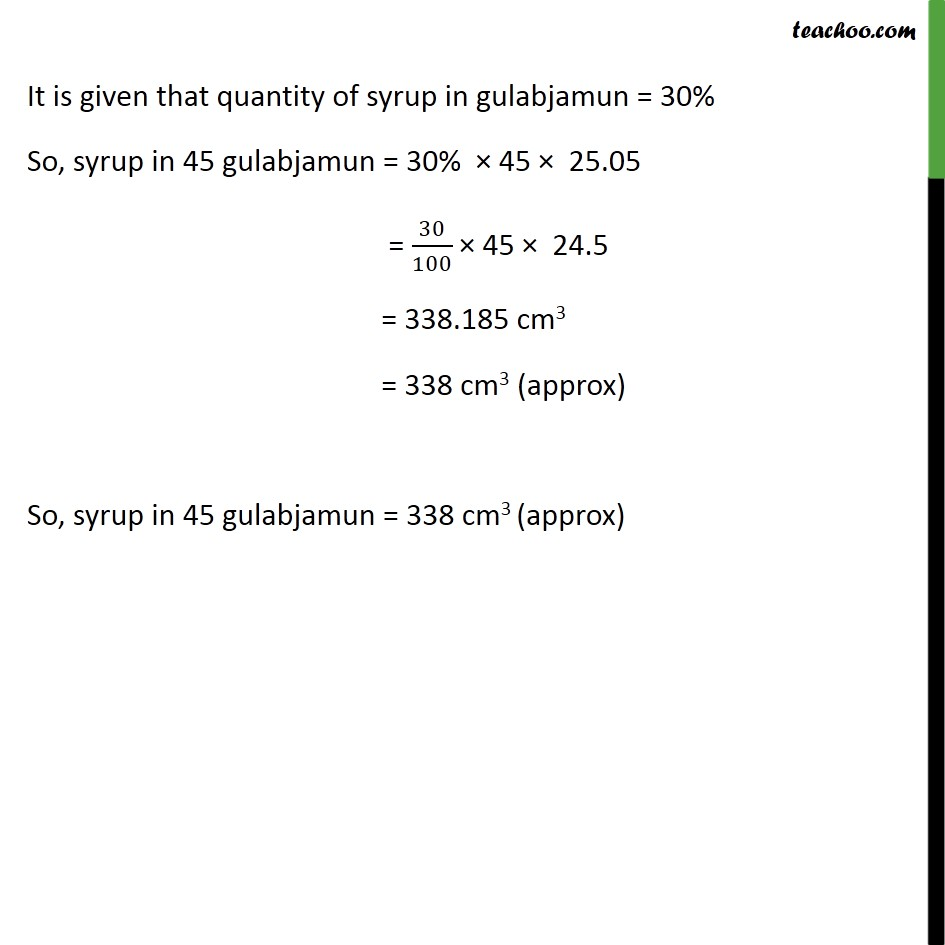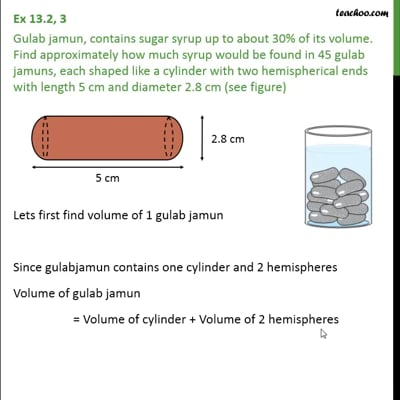This video is only available for Teachoo black users

### Transcript

Ex 13.2, 3 Gulab jamun, contains sugar syrup up to about 30% of its volume. Find approximately how much syrup would be found in 45 gulab jamuns, each shaped like a cylinder with two hemispherical ends with length 5 cm and diameter 2.8 cm (see figure) Lets first find volume of 1 gulab jamun Since gulabjamun contains one cylinder and 2 hemispheres Volume of gulab jamun = Volume of cylinder + Volume of 2 hemispheres Volume of cylinder Diameter of cylinder = 2.8 cm Radius of cylinder = ( )/2 = 2.8/2 = 1.4 cm Here, Diameter of hemisphere = 2.8 cm So, Radius of hemisphere = ( )/2 = 2.8/2 = 1.4 cm Now, Height of cylinder + 2 Radius of hemisphere = 5 cm Height of cylinder = 5 2 Radius of hemisphere = 5 2 1.4 = 5 2.8 = 2.2 cm Volume of cylinder = 2 = 22/7 (1.4)2 2.2 = 22/7 1.4 1.4 2.2 = 22 0.2 1.4 2.2 = 13.55 cm3 Volume of hemisphere Radius of hemisphere = 1.4 cm Volume of hemisphere = 2/3 r3 = 2/3 22/7 (1.4)3 = 2/3 22/7 1.4 1.4 1.4 = 5.75 cm3 Volume of 2 hemispheres = 2 Volume of hemisphere = 2 5.75 = 11.50 cm3 Now, Volume of gulab jamun = Volume of cylinder + Volume of 2 hemispheres = 13.55 + 11.50 = 25.05 cm3 So, volume of 1 gulabjamun = 25.05 cm3 Volume of 45 gulabjamun = 45 25.05 cm3 We need to find quantity of syrup in 45 gulabjamun It is given that quantity of syrup in gulabjamun = 30% So, syrup in 45 gulabjamun = 30% 45 25.05 = 30/100 45 24.5 = 338.185 cm3 = 338 cm3 (approx) So, syrup in 45 gulabjamun = 338 cm3 (approx)